Courses

# Important Questions: Symmetry

## 20 Questions MCQ Test Mathematics (Maths) Class 7 | Important Questions: Symmetry

Description
This mock test of Important Questions: Symmetry for Class 7 helps you for every Class 7 entrance exam. This contains 20 Multiple Choice Questions for Class 7 Important Questions: Symmetry (mcq) to study with solutions a complete question bank. The solved questions answers in this Important Questions: Symmetry quiz give you a good mix of easy questions and tough questions. Class 7 students definitely take this Important Questions: Symmetry exercise for a better result in the exam. You can find other Important Questions: Symmetry extra questions, long questions & short questions for Class 7 on EduRev as well by searching above.
QUESTION: 1

### Which of the following alphabets has a vertical line of symmetry?

Solution:

Recall definition of line symmetry.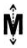QUESTION: 2

### Which of the following alphabets has a horizontal line of symmetry?

Solution:

All of the letters have horizontal line of symmetry since it cut them in equal parts if we draw a line in the middle of each of the letters.

QUESTION: 3

### Which of the following alphabets has no line of symmetry?

Solution:

P has no line of symmetry.

QUESTION: 4

Which of the following alphabets has many lines of symmetry?

Solution:

O has many lines of symmetry.

QUESTION: 5

Which of the following triangles has no line of symmetry?

Solution:

A scalene triangle has no line of symmetry.

QUESTION: 6

What is the mirror image of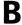in a horizontal mirror?

Solution:

Since horizontal mirror is mirror along with the x-axis , so B will be seen as B

QUESTION: 7

Which of the following figures are symmetrical with respect to exactly two lines?

Solution:

Only the third figure has horizontal and vertical symmetry. So it has 2 lines of symmetry.

QUESTION: 8

A Which of the following figures has rotational symmetry of order more than 1?

Solution:

Rotational Symmetry is how many times a shape fits into itself when rotated.So when a circle is rotated by 90 degree it fits into its original space. So its order is 4 since it fits into itself 4 times. An equilateral triangle when rotated by 120 degrees fits into itself. So its order is 3. The 3rd shape rotates the same as the circle. So its order is 4.

QUESTION: 9

A What is the order of rotational symmetry for the given figure?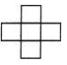Solution:
QUESTION: 10

What is the order of rotational symmetry for the given figure?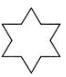Solution:
QUESTION: 11

Which of the following figures has only one line of symmetry?

Solution:

The second figure has only the vertical line of symmetry. So it has only 1 line of symmetry.

QUESTION: 12

Identify the number of lines of symmetry for the figure given.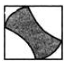Solution:

There are only two possibilities i.e., diagonal lines of symmetry. So there are two lines of symmetry.

QUESTION: 13

In the given figure, the dotted line is the line of symmetry. Which figure is formed if the given figure is reflected in the dotted line?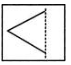Solution: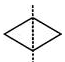The figure formed is a rhombus.

QUESTION: 14

What is the other name for a line of symmetry of a circle?

Solution:

A diameter divides the circle into 2 equal parts. So, it can be considered as a line of symmetry.

QUESTION: 15

A In A XYZ, XY = XZ and XM⊥YZand ZP⊥XY. About which of the following is the triangle symmetrical?

Solution: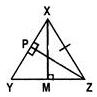Since XY=XZ and XM⊥YZ,

∠YXM=∠ZXM. So, the triangle is symmetrical about XM as it bisects the apex angle.

QUESTION: 16

What is the order of rotational symmetry of the English alphabet Z?

Solution:
QUESTION: 17

Which of these quadrilaterals have both line and rotational symmetries of order more than 3?

Solution:
QUESTION: 18

Which of these letters has only rotational symmetry?

Solution:

Rotational symmetry in capital letters describes a property in which the letter looks the same after being rotated. Capital letters that have rotational symmetry are: Z, S, H, N and O.
The letters Z, S, H and N, when rotated 180 degrees clockwise or counterclockwise, will look the same after rotation completion. This is called rotational symmetry on the order of two, because there were two 90 degree rotations. The letter O has rotational symmetry on the order of one, because after being rotated by 90 degrees, it still looks the same.

QUESTION: 19

A square has a rotational symmetry of order 4 about its centre. What is the angle of rotation?

Solution:
QUESTION: 20

What is the order of rotational symmetry of the figure given?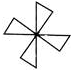Solution: